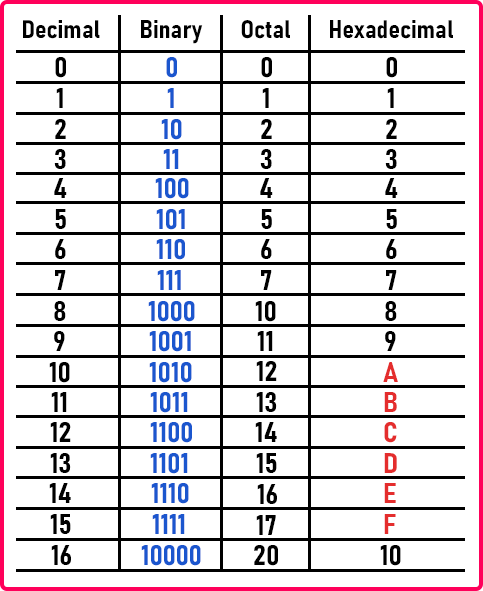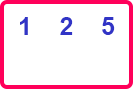# Octal and Hexadecimal to Binary Conversion

## Octal and Hexadecimal to Binary Conversion:

In this article, I am going to discuss Octal and Hexadecimal to Binary Conversion as well as Binary to Octal and Hexadecimal Conversion with Examples. Please read our previous article where we discussed Binary, Octal, Hexadecimal to Decimal Conversion with Examples.

##### Octal and Hexadecimal to Binary Conversion:

We will see how to convert octal numbers to binary and vice versa. Similarly, we look for a hexadecimal number system. For that, we have to observe something from the following table.##### Octal to Binary Conversion:

Let us look at the octal numbers in the above table. If you observed the octal numbers, there is the 2-digit number at the 8th position which is 10. And if you observe it in binary, this is becoming a 4-digit number at the 8th position. So, digits are increasing. From 0 to 7 in octal numbers, 3 digits of binary are sufficient. So, by taking this benefit we can directly convert octal to binary and binary to octal. So let us see how.

Suppose we have an octal number 125. This 125 is an octal number, not a decimal number. This is a figure, not the value. Let us convert it into binary. First, we have to write the digits as,Now, we know that 5 in the octal number system is equivalent to 101. So let us write it as,In the same manner, we have to write 1 and 2 of octal number in binary number as,Here 1 is of 1 digit and 10 is of 2 digits. But we have to write these as 3 digits.So, we have written all the binary numbers in 3 digits by leading zeros. Now let us form the binary number with these binary digits sequence. As it is starting with 2 zeros and only 0 has no value so let us remove those zeros.

1010101

So, the number is formed by writing these binary digits from left to right order. So,

(125)8 = (1010101)2

Now let us take another number, 274. This is in an octal number. Let us convert this into a binary number system. So first we have to write these octal digits.Now let us write these digits in binary form. You can take the help of the above table.Now again we have to combine these digits from left to right order. So, the number is 10111100

(274)8= (10111100)2

We know very well that 3 digits of binary form are single digits of octal form. So, we just write the octal digits separately, and then below every digit, we have written a bundle of 3 digits of binary form. And lastly, we just wrote the digits in left to right order.

##### Binary to Octal Conversion:

Now let us convert (10111100)2 into octal form. Here we will make a bundle of 3 digits from the right-hand side.We have bundled the pair of 3 digits. Now we will convert these binary pairs to octal numbers. We know that,

(010)2 = (2)8

(111)2 = (7)8

(100)2 = (4)8

Let us replace the above binary pairs with the octal numbers,(10111100)2 = (274)8

So, this is in octal form. This is how we can convert a binary number to an octal number. Similarly, now we will learn the conversion of hexadecimal numbers to a binary number and vice versa.

Let us again observe the table. In hexadecimal, a figure is a digit number at position 16. If you observe binary figures, up to value 15 it is a 4-digit number and at 16 it is a 5-digit number. With this observation, we can say that four digits are required for a hexadecimal number. In octal, 3 digits of binary are required and in hexadecimal, 4 digits of binary are required. Let us take a hexadecimal number and convert that into binary. The number is 2A. So first we have to write these digits separately,Here, 2 in hexadecimal is equivalent to 0010 in binary and A is equivalent to 1010.So, the binary form is 00101010. We can neglect the starting zeroes as they have no value. So the figure is 101010.

(2A) 16 = (101010)2

Let us take one more hexadecimal number, 7C4. First, we will write these digits separately,Now we will write their corresponding binary digits.See here 4 of hexadecimal is equivalent to 100 in binary. This is a 3-digit figure. But we want 4. So, we have to make it a 4-digit figure by leading it with zeros. We can’t neglect 0 here because this is not the first digit of the figure. We can neglect leading zeros in only the first digit of the hexadecimal figure i.e. 7. Now the figure in decimal is,

(7C4) 16 = (11111000100)2

So, in the hexadecimal system, we have to replace every digit with 4 digits in binary. This is all about the conversion of hexadecimal to the binary number system. Now let us see the binary to the hexadecimal number system.

Let us take the number 101010. Now make a bundle of 4 digits,Now convert these underlined figures into hexadecimal digits with the help of the above table.(101010)2 = (2A) 16

So, the converted hexadecimal figure is 2A. Let us take one more binary number which is 10011001010. So again, we will this figure as the bundle of 4 digits.Now we will write hexadecimal digits,(10011001010)2 = (4A) 16